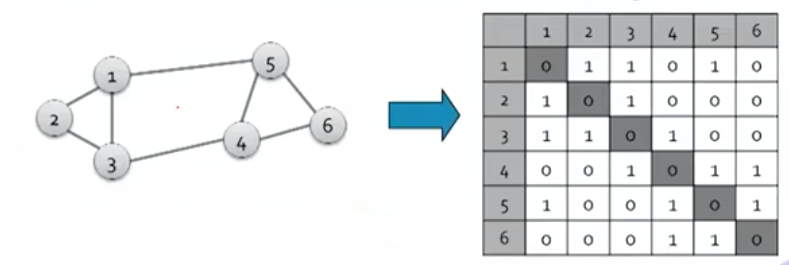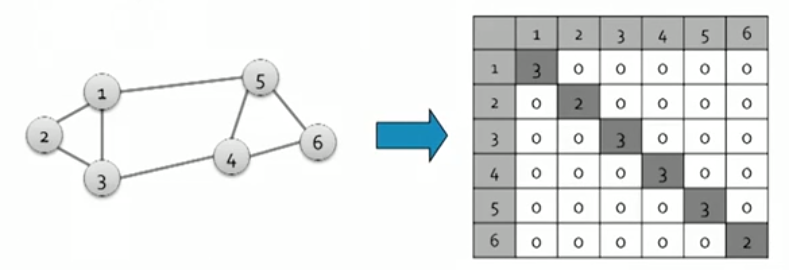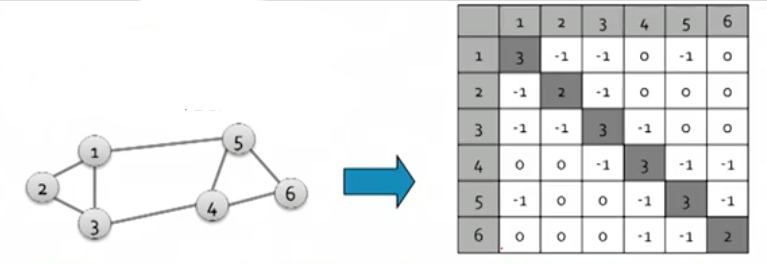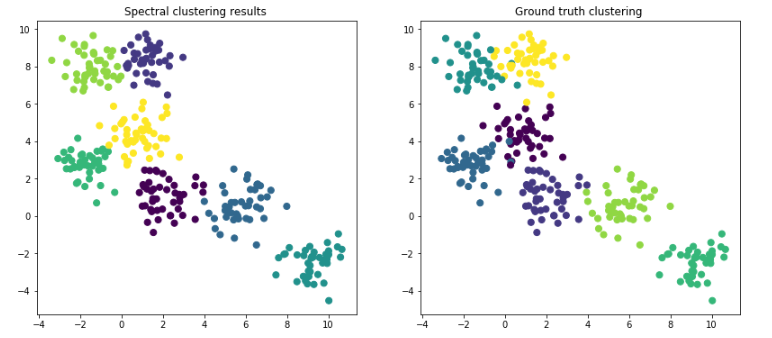Search
• Madalina Ciortan

# Spectral clustering demystified

Let’s start by introducing some basing graph theory notions.

### Adjacency matrix (A)

Given a graph with n vertices and m nodes, the adjacency matrix is a square n*n matrix with the property:

A[i][j] = 1 if there is an edge between node i and node j, 0 otherwiseBecause A is symmetric, its eigen vectors are real and orthogonal (the dot product is 0).

### Degree matrix (D)

The degree matrix is a n*n diagonal matrix with the property

d[i][i] = the number of adjacent edges in node i or the degree of node i

d[i][j] = 0### Laplacian matrix (L)

The laplacian matrix is a n*n matrix defined as: L = D -A

Its eigen values are positive real numbers and the eigen vectors are real and orthogonal (the dot product of the 2 vectors is 0)### Conductance

A measure of the connectivity of a group to the rest of the network relative to the density of the group (the number of edges that point outside the cluster divided by the sum of the degrees of the nodes in the cluster). The lower the conductance, the better the cluster.

Calculating the eigen values and eigen vectors of A with x ( n dimensional vector with the values of the nodes): A * x = lambda * x

The spectrum of a matrix representing the graph G is a set of eigenvectors xi of the graph ordered by the corresponding eigen values lambda i.

Now that we introduced the most important building blocks of graph theory, we are ready to summarize the spectral clustering steps:

Compute the Laplacian matrix L of the input graph Compute the eigen values (lambda) and eigen vectors (x) such that

L* x = lambda * x

3. Select n eigenvectors corresponding to the largest eigenvalues and redefine the input space as a n dimensional subspace

4. Find clusters in this subspace using various clustering algorithms, such as k-means

It is also possible to use instead of the adjacency matrix defined above an affinity matrix which determines how close or similar are 2 points in our space. As defined in the sklearn implemenatation

`similarity = np.exp(-beta * distance / distance.std()) `

A good resource demoing the creation of the affinity matrix is this youtube video.

Both Spectral Clustering and affinity propagation have been implemented in python. This jupiter notebook shows a quick demo of their usage.

```clustering = SpectralClustering(n_clusters=nb_clusters, assign_labels="discretize", random_state=0).fit(X)
y_pred = clustering.labels_
plt.title(f'Spectral clustering results ')
plt.scatter(X[:, 0], X[:, 1], s=50, c = y_pred);```### Self tuning Spectral Clustering

The idea behind the self tuning spectral clustering is to avoid giving as input to the spectral clustering algorithm the number of clusters. This can be achieved either by changing the way the affinity matrix is being built by putting a constraint on the number of nearest neighbors or by determining from the structure of the eigenvectors the optimal number of clusters.

See All